# Plus One Maths Chapter Wise Questions and Answers Chapter 8 Binomial Theorem

Students can Download Chapter 8 Binomial Theorem Questions and Answers, Plus One Maths Chapter Wise Questions and Answers helps you to revise the complete Kerala State Syllabus and score more marks in your examinations.

## Kerala Plus One Maths Chapter Wise Questions and Answers Chapter 8 Binomial Theorem

### Plus One Maths Binomial Theorem Three Mark Questions and Answers

Question 1.
Write the middle term in the expansion of the following; (3 score each)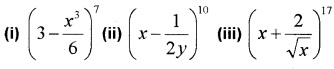i) Here 7 is an odd number. Therefore there are two middle terms $$\left(\frac{7+1}{2}=4\right)^{t h}$$ and $$\left(\frac{7+1}{2}+1=5\right)^{t h}$$, ie; 4th and 5th terms in the above expansion.ii) Here 10 is an even number. Therefore middle terms $$\left(\frac{10}{2}+1=6\right)^{t h}$$ term in the above expansion.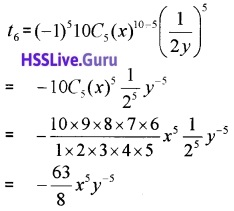iii) Here 17 is an odd number. Therefore there are two middle terms $$\left(\frac{17+1}{2}=9\right)^{t h}$$, ie; 9th and 10th terms in the above expansion. $$\left(x+\frac{2}{\sqrt{x}}\right)^{17}$$.Question 2.
Find the term independent of x in the following expansion. (3 score each)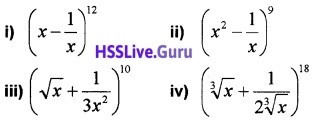i) General term = tr+1 = (-1)r12Cr(x)12-r $$\left(\frac{1}{x}\right)^{r}$$
=(-1)r12Cr(x)12-r-r = (-1)r12Cr(x)12-2r
Term independent of x in the expansion will be the term in which the power of x is zero, ie; 12 – 2r = 0 ⇒ 12 = 2r
⇒ r = 6
t7 = (-1)612C6x12-2(6)
= $$\frac{12 \times 11 \times 10 \times 9 \times 8 \times 7}{1 \times 2 \times 3 \times 4 \times 5 \times 6}$$ = 924.

ii) General term = tr+1 =(-1)r9Cr(x2)9-r$$\left(\frac{1}{x}\right)^{r}$$
= (-1)r9Cr(x)18-2r-r = (-1)r9Cr(x)18-3r
Term independent of x in the expansion will be the term in which the power of x is zero.
ie; 18 – 3r = 0 ⇒ 18 = 3r ⇒ r = 6
t7 = (-1)69C6x18-3(6)
= $$\frac{9 \times 8 \times 7}{1 \times 2 \times 3}$$ = 84.

iii) General term = tr+1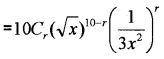Term independent of x in the expansion will be the term in which the power of x is zero.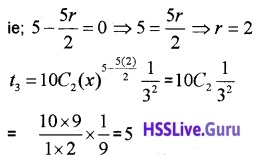iv) General term = tr+1Term independent of x in the expansion will be the term in which the power of x is zero.Question 3.
Find the coefficient of x10 in the expansion of $$\left(2 x^{2}-\frac{3}{x}\right)^{11}$$.
General term = tr+1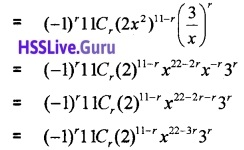Given; 22 – 3r = 10 ⇒ 12 = 3r ⇒ r = 4
t5 = (-1)411C4211-4 x22-3(4) 34
= 11C42734x10
Therefore the coefficient of x10 is 11C42734.Question 4.
Find the coefficient of a5b7 in the expansion of (a – 2b)12.
General term = tr+1 = (-1)r12Cr(a)12-r(2b)r
= (-1)r12Cr(a)12-r2rbr
The term containing a5b7 is obtained by putting r = 7
⇒ t8 = (-1)712C7(a)12-727b7
Therefore the coefficient of a5b7 is
(-1)712C727 = -12C727.

Question 5.
Find the coefficient of (3 score each)

1. x11 in the expansion of $$\left(x-\frac{2}{x^{2}}\right)^{17}$$
2. x9 in the expansion of $$\left(3 x^{2}+\frac{5}{x^{3}}\right)^{12}$$
3. x20 in the expansion of $$\left(3 x^{3}-\frac{2}{x^{2}}\right)^{40}$$

1. General termThe term containing x11 is obtained by
17 – 3r = 11 ⇒ 6 = 3r ⇒ r = 2
⇒ t3 = (-1)217C2 (x)17-3(2) 22 = 17C2(x)11 × 4
Therefore the coefficient of x11 is 17C2 × 4
= $$\frac{17 \times 16}{1 \times 2}$$ × 4 = 544

2. General termThe term containing x9 is obtained by
24 – 5r = 9 ⇒ 15 = 5r ⇒ r = 3
⇒ t4 = 12C3(3)12-3(x)24-5(3)53
= 12C3(3)9(x)953
Therefore the coefficient of x9 is 12C3(3)953.

3. General term = tr+1
= (-1)r40Cr(3x3)40-r ($$\frac{2}{x^{2}}$$)r
= (-1)r40Cr(3)40-rx120-3r (2)r x-2r
= (-1)r40Cr(3)40-rx120-5r(2)r
The term containing x20 is obtained by
120 – 5r = 20 ⇒ 100 = 5r ⇒ r = 20
⇒ t21 = (-1)2040C20(3)40-20(x) 120-5(20) 220
= 40C20(3)20(x)20220
Therefore the coefficient of x20 is 40C20(3) 20 220.Question 6.

1. Find the term independent of x in the expansion of $$\left(x^{2}+\frac{2}{x}\right)^{6}$$ (3)
2. If the middle term in the expansion of $$\left(x^{m}+\frac{2}{x}\right)^{6}$$ is independent of x, find the value of m.

1. tr+1 = nCran-rbr = 6Cr(x2)6-r$$\left(\frac{2}{x}\right)^{r}$$
= 6Crx12-2rx-r(2)r = 6Crx12-3r(2)r
For term independent of x;
12 – 3r = 0 r = 4
t5 = 6C4(2)4 = 6C2 × 16 = $$\frac{6 \times 5}{1 \times 2}$$ × 16 = 240

2. m = 1

### Plus One Maths Binomial Theorem Four Mark Questions and Answers

Question 1.

1. Write the general term in the expansion $$\left(\frac{3 x^{2}}{2}-\frac{1}{3 x}\right)^{6}$$ (2)
2. Find the term independent of x in the above expansion. (2)

1. General term = tr+12. Term independent of x in the expansion will be the term in which the power of x is zero.
ie; 12 – 3r = 0 ⇒ 12 = 3r ⇒ r = 4### Plus One Maths Binomial Theorem Practice Problems Questions and Answers

Question 1.
Expand the following. (2 score each)

1. (3a2 – 2b)4
2. (3 – 4x2)5
3. $$\left(\frac{x}{2}-2 y\right)^{6}$$
4. $$\left(\frac{x}{2}-2 y\right)^{6}$$

1.2.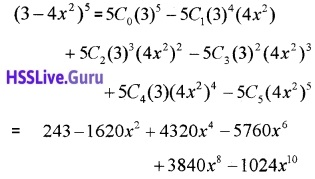3.4.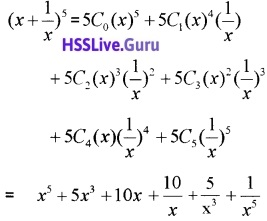Question 2.
Write the general term in the expansion of the following; (2 score each)i) General term = tr+1
= (-1)r6Cr(x2)6-r(y)r
= (-1)r6Crx12-ryr.

ii) General term = tr+1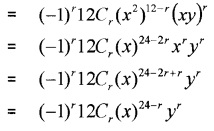iii) General term = tr+1iv) General term = tr+1Question 3.
If the coefficient of x2 in the expansion of (1 + x)n is 6 then the positive value of n.
nC2 = 6 ⇒ $$\frac{n(n-1)}{2}$$ = 6 ⇒ n(n -1) = 12
Find the 13th term in the expansion of $$\left(9 x-\frac{1}{3 \sqrt{x}}\right)^{18}$$.